# Bihar Board Class 10 Science Solutions Chapter 5 Periodic Classification of Elements

BSEB Bihar Board Class 10 Science Solutions Chapter 5 Periodic Classification of Elements Textbook Questions and Answers.

## Bihar Board Class 10 Science Solutions Chapter 5 Periodic Classification of Elements

### Bihar Board Class 10 Science Chapter 5 Periodic Classification of Elements InText Questions and Answers

In-text Questions (Page 81)

Question 1.
Did Dobereiner’s triads also exist in the columns of Newlands’ Octaves? Compare and find out.
Yes, some of the Dobereiner’s triads did exist in the columns of Newland’s octaves.
For example,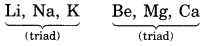Question 2.
What were the limitations of Dobereiner’s classification?
Limitations of Dobereiner’s classification:

• Dobereiner could not classify all the elements known at that time.
• He could identify only three triads from the elements known at that time.
• He failed to arrange all the elements in the form of triads having similar chemical properties.
• The system of classification into triads was not accepted.

Question 3.
What were the limitations of Newlands’ Law of Octaves?
Limitations of the Law of Octaves:

1. It was found that this law was applicable only upto the element calcium (Ca), as after calcium every eight elements did not possess properties similar to that of the first.
2. It was assumed by Newlands that only 56 elements existed in nature and no more elements would be discovered in the future. But many more new elements were discovered and their properties did not fit into the law of octaves.
3. In order to give place to the new elements, Newlands adjusted two elements in the same slot but their properties did not agree with each other.

In-text Questions (Page 85)

Question 1.
Use Mendeleev’s Periodic Table to predict the formulae for the oxides of the following elements:
K, C, Al, Si, Ba

• Potassium (K) is an element of group IA (alkali metals). Its valency is 1. Therefore, the formula of its oxide is K2O.
• Carbon (C) is an element of group IVA. Its valency is 4. Therefore, the formula of its oxide is CO2.
• Aluminium (Al) is an element of group IIIA. Its valency is 3. Therefore, the formula of its oxide is Al2O3.
• Silicon (Si) is an element of group IVA. Its valency is 4. Therefore, the formula of its oxide is SiO2.
• Barium (Ba) is an element of group IIA. Its valency is 2. Therefore, the formula of its oxide is BaO.

Question 2.
Besides gallium, which other elements have since been discovered that were left by Mendeleev in his Periodic Table? (any two)
Two other elements are scandium (Sc) and germanium (Ge). In their gaps, the elements with names Eka-boron and Eka-silicon were placed.

Question 3.
What were the criteria used by Mendeleev in creating his Periodic Table?

• Mendeleev arranged the elements on the basis of their increasing atomic masses.
• The elements with similar properties could be grouped together.
• He selected hydrogen and oxygen as they are very reactive and formed compounds with most elements. The formulae of the oxides and hydrides formed by an element were treated as one of the properties of an element for its classification.

Question 4.
Why do you think the noble gases are placed in a separate group?
Out of all the elements known, noble gases such as helium (He), neon (Ne), argon (Ar), krypton (Kr) and xenon (Xe) are the most inert (unreactive) and are present in extremely low concentrations in our atmosphere. Therefore, they are grouped together in a separate group called zero group in the modified Mendeleev’s Periodic Table.

In-text Questions (Page 90)

Question 1.
How could the Modern Periodic Table remove various anomalies of Mendeleev’s Periodic Table?
Modern Periodic Table is based on the atomic number of the atoms of elements. As we know that the properties of the elements depend on valence electrons present in the outermost shell of its atoms, the distribution of electrons depends on the atomic number. So on the basis of atomic number even the isotopes of an element will be given one position in the Modern Periodic Table. Modern Periodic Table is able to remove various anomalies of Mendeleev’s Periodic Table.

(i) Position of Isotopes: All the atoms of an element have the same number of protons, so their atomic number is also same. Since all the isotopes of an element have the same atomic number, they can be put at one place in the same group of the periodic table.

(ii) Position of Cobalt and Nickel: In Mendeleev’s Periodic Table, cobalt with slightly higher atomic mass was placed before nickel with slightly lower atomic mass. Atomic number of cobalt is 27 and that of nickel is 28. Since Modern Periodic Table is based on atomic number so it is proved to be right.

(iii) Position of Hydrogen: Hydrogen has been placed at the top of Group 1, above the alkali metals because the electronic configuration of hydrogen is similar to that of alkali metals. Both, hydrogen as well as alkali metals have 1 valence electron each.

Question 2.
Name two elements you would expect to show chemical reactions similar to magnesium. What is the basis for your choice?
According to Modern Periodic Table, elements having similar outer electronic configuration show similar properties. Since magnesium has two electrons in the valence shell (outermost shell), therefore, all the elements having two electrons in the valence shell show similar properties. For example,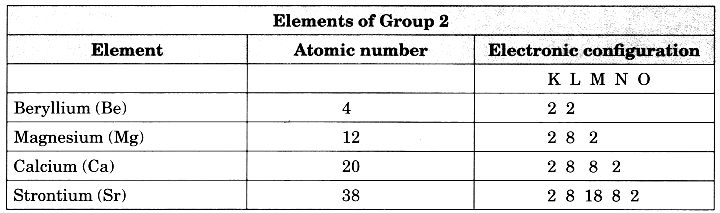Thus, elements of group 2 such as beryllium (Be), calcium (Ca), strontium (Sr), etc. show similar properties.

Question 3.
Name:
(a) three elements that have a single electron in their outermost shells.
(b) two elements that have two electrons in their outermost shells.
(c) three elements with filled outermost shells.
(a) Lithium—Li, Sodium—Na, Potassium—K
(b) Beryllium—Be, Magnesium—Mg, Calcium—Ca
(c) Helium—He, Neon—Ne, Argon—Ar

Question 4.
(a) Lithium, sodium, potassium are all metals that react with water to liberate hydrogen gas. Is there any similarity in the atoms of these elements?
(b) Helium is an unreactive gas and neon is a gas of extremely low reactivity. What, if anything, do their atoms have in common?
(a) Yes, the atoms of all the three elements- lithium, sodium, and potassium have one electron in their outermost shells.
(b) Both helium (He) and neon (Ne) have filled outermost shells. Helium has a duplet in its K shell, while neon has an octet in its L shell.

Question 5.
In the Modern Periodic Table, which are the metals among the first ten elements?
Among the first ten elements, lithium (Li) and beryllium (Be) are metals.

Question 6.
By considering their position in the Periodic Table, which one of the following elements would you expect to have maximum metallic characteristics?
Ga Ge As Se Be
Since Be lies to the extreme left hand side of the periodic table, Be is the most metallic among the given elements.

### Bihar Board Class 10 Science Chapter 5 Periodic Classification of Elements Textbook Questions and Answers

Question 1.
Which of the following statements is not a correct statement about the trends when going from left to right across the periods of periodic Table.
(a) The elements become less metallic in nature.
(b) The number of valence electrons increases.
(c) The atoms lose their electrons more easily.
(d) The oxides become more acidic.
(c) The atoms lose their electrons more easily.

Question 2.
Element X forms a chloride with the formula XCl2, which is a solid with a high melting point. X would most likely be in the same group of the Periodic Table as
(a) Na
(b) Mg
(c) Al
(d) Si
(b) Mg

Question 3.
Which element has
(a) two shells, both of which are completely filled with electrons?
(b) the electronic configuration 2, 8, 2?
(c) a total of three shells, with four electrons in its valence shell?
(d) a total of two shells, with three electrons in its valence shell?
(e) twice as many electrons in its second shell as in its first shell?
(a) Ne (2, 8)
(b) Mg
(c) Si (2, 8, 4)
(d) B (2, 3)
(e) C (2, 4)

Question 4.
(a) What property do all elements in the same column of the Periodic Table as boron have in common?
(b) What property do all elements in the same column of the Periodic Table as fluorine have in common?
(a) The element boron is in column (or group) 13 of the periodic table and has a valency of 3. So, all the elements in the same column of Periodic table as boron will have valency of 3.
(b) The elements fluorine is in column (or group) 17 of the Periodic table and has a valency of 1. So, all the elements in the same column of Periodic Table as fluorine will have a valency of 1.

Question 5.
An atom has electronic configuration 2, 8, 7.
(a) What is the atomic number of this element?
(b) To which of the following elements would it be chemically similar? (Atomic numbers are given in parentheses.)
N(7) F(9) P(15) Ar(18)
(a) Its atomic number is 17 (equal to total number of electrons present in all its shells, i.e., 2 + 8 + 7 = 17)
(b) Electronic configuration of the given elements is 2, 8, 7.

Electronic configuration of these elements are:
N = 2, 5
F = 2, 7
P = 2, 8, 5
Ar = 2, 8, 8

The properties of these elements are similar to F (fluorine) element as both have same number of valence electrons (electrons in the outermost shell.)

Question 6.
The position of three elements A, B and C in the Periodic Table are shown below: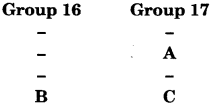(a) State whether A is a metal or non-metal.
(b) State whether C is more reactive or less reactive than A.
(c) Will C be larger or smaller in size than B?
(d) Which type of ion, cation or anion, will be formed by element A?
(a) Elements of group 17 have 7 electrons in their respective valence shells and thus have a strong tendency to gain one more electron to complete their respective octets. Thus, C which is an element of group 17 is a non-metal.

(b) As we move down the group, the atomic size increases. Therefore, the force of attraction of the nucleus for the incoming electron decreases and hence the reactivity decreases down the group. Since element C has larger atomic size than element A, therefore, element C is less reactive than element A.

(c) Elements B and C belong to the same period, i.e., 5th period. As we move from left to right in a period, the nuclear charge increases but the number of shells remains the same. Therefore, the size decreases from left to right. Thus, the atomic size of C is smaller than that of B.

(d) Since element A has 7 electrons in the valence shell, therefore, it has a high tendency to gain one electron to complete its octet. Thus, element A forms an anion, i.e.,
$$\underset{\text { Atom }}{\mathrm{A}}+\underset{\text { Electron }}{e^{-}} \longrightarrow \underset{\text { Anion }}{\mathrm{A}^{-}}$$

Question 7.
Nitrogen (atomic number 7) and phosphorus (atomic number 15) belong to group 15 of the Periodic Table. Write the electronic configuration of these two elements. Which of these will be more electronegative? Why?
The electronic configuration of the two elements are:
Nitrogen (z = 7) 2, 5; phosphorus ( z = 15) 2, 8, 5
Since the size of nitrogen is small as compared to phosphorus, it has a greater tendency to take up electrons. It is therefore, more electronegative than phosphorus.

Question 8.
How does the electronic configuration of an atom relate to its position in the Modern Periodic Table?
Electronic configuration of an element tells two things:

• Number of electrons in the outermost shell.
• Number of shells having the electrons.

The position of an atom or element in the periodic table is related to the number of electrons in the outermost shell. In a given group, all elements have the same number of electrons in their outermost shells.

For example, In group 2, all elements have two electrons in their outermost shell. In group 15, all elements have 5 elements in their outermost shells.

Question 9.
In the Modern Periodic Table, calcium (atomic number 20) is surrounded by elements with atomic numbers 12, 19, 21 and 38. Which of these have physical and chemical properties resembling calcium?
Atomic number of calcium = 20 ∴ Electronic configuration = 2, 8, 8, 2
Calcium surrounded by elements:
(i) Atomic number = 12 ∴ Electronic configuration = 2, 8, 2
(ii) Atomic number = 19 ∴ Electronic configuration = 2, 8, 8, 1
(iii) Atomic number = 21 ∴ Electronic configuration = 2, 8, 8, 3
(iv) Atomic number = 38 ∴ Electronic configuration = 2, 8, 18, 8, 2
The elements having atomic number 12 (magnesium: Mg) and atomic number 38 (strontium: Sr) resemble the properties of calcium (Ca).

Question 10.
Compare and contrast the arrangement of elements in Mendeleev’s Periodic Table and the Modern Periodic Table.
The main points of distinction between Mendeleev’s periodic table and Modern periodic table are as follows:
Mendeleev’s Periodic Table:

1. It regards atomic masses of the elements as the basis of classification.
2. No separate positions or slots have been allotted to the isotopes of an element since they have different atomic masses.
3. No justification is made for placing hydrogen in group IA along with alkali metals.
4. Except for the elements in group VIII, the remaining groups have been divided with sub-groups A and B.
5. Position of certain elements based on their atomic masses are misfits. For example, the element cobalt (atomic mass = 58.9) has been its placed ahead of nickel (atomic mass = 58.7).
6. Electronic configurations and properties of the elements cannot be predicted from their positions in the table.
7. It is not very systematic and is difficult to remember.

Modem Periodic Table

1. It regards atomic number of the elements as the basis of classification.
2. There is no need for the separate slots for the isotopes since they have the same atomic numbers.
3. Justification has been made for placing hydrogen along with alkali metals in group 1 since both hydrogen and alkali metals have one valence electron.
4. There are no sub-groups and all groups are independent in nature.
5. Modern periodic table is free from such anomalies. The element cobalt is placed before nickel since its atomic number (27) is less than that of nickel (28).
6. Both electronic configuration and certain properties of the elements can be predicted from their positions in the periodic table.
7. It is quite systematic and is easy to remember.

### Bihar Board Class 10 Science Chapter 5 Periodic Classification of Elements Textbook Activities

Activity 5.1 (Textbook Page 84)

Observation: Hydrogen has the lower atomic number (Z = 1) and lowest atomic mass (1.008 u). Obviously it is placed in group 1 and period 1. Since the properties of hydrogen resemble both alkali metals and halogens, therefore, it can be placed either along with alkali metals of group I A or halogens of group VII A of the Mendeleev’s periodic table.

Conclusion: No correct position can be assigned to hydrogen in the periodic table. In other words, position of hydrogen in the periodic table is anomalous or controversial. Usually it is placed in group 1 and period 1 because it has the lowest atomic number (i.e. 1) and has the lowest atomic mass (1.008 u).

Activity 5.2 (Textbook Page 85)

Observation: In the Mendeleev’s periodic table, elements are arranged in order of their increasing atomic masses. Therefore, in principle, the two isotopes of chlorine, i.e., Cl – 35 and Cl – 37 should be placed at different positions because their atomic masses (i.e., 35u and 37u) are different but there is no vacant position available for Cl – 37 in between Cl – 35 of group VIIA and K(39.1 u) of group IA.

However, in the long form of the periodic table, elements are arranged in order of their increasing atomic numbers. Therefore, the two isotopes of chlorine, i.e., Cl – 35 and Cl – 37 have the same atomic number (z = 17) and hence have similar chemical properties. Thus, they can be placed at the same position in group 17 of the long form of the periodic table.

Conclusion: Since isotopes have the same atomic number, therefore, they are placed at the same position in a particular group of the long form of the periodic table.

Activity 5.3 (Textbook Page 85)

Observation:

• In modern periodic table, elements are placed in increasing order of their atomic numbers. Since atomic number of Co is 27 and that of Ni is 28, therefore, cobalt (group 9) is placed before nickel (group 10) and hence their positions are justified in the Modern Periodic Table.
• In the Modern Periodic Table, elements are arranged in increasing order of their atomic number. Since the various isotopes of an element have the same atomic number, therefore, they are assigned the same position in the Modern Periodic Table.
• An element with atomic number 1.5 is not possible because the atomic numbers is always in whole numbers.
• Hydrogen should be placed in Period 1 and group 1 in the Modern Periodic Table.

Activity 5.4 (Textbook Page 87)

Observation and conclusions:
The names of the elements present in group 1 are: hydrogen (H), lithium (Li), sodium (Na), potassium (K), rubidium (Rb), caesium (Cs) and francium (Fr).

Electronic configuration of elements are: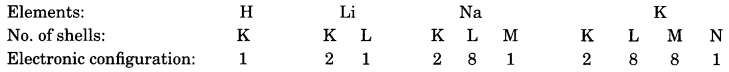All these elements have one electron in their respective valence shells.

Activity 5.5 (Textbook Page 87)

Observation:
The electronic configurations of the elements of second period are: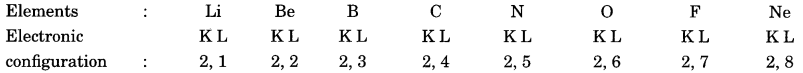• These elements do not contain the same number of valence electrons. The number of valence electrons actually increases from 1 to 8.
• All these elements contain the same number of shell, i.e., two, K and L.

Activity 5.6 (Textbook Page 87)

Observation:
For elements of group 1, 2, 13 and 14 the valency is equal to the number of valence electrons and for elements of group 15 to 18, the valency is equal to 8 – No. valence electrons.

Electronic configuration of Mg with atomic number 12 is
K L M
2 8 2
Therefore, the number of electrons in the valence shell = 2.
Since the element belongs to group 2, therefore, its valency is equal to the number of electrons in the valence shell = 2.
The electronic configuration of sulphur with atomic number 16 is
K L M
2 8 6

Therefore, the number of electrons in the valence shell = 6
Since the element belongs to group 16, therefore, its valency = 8 – 6 = 2.
Please note that, in case of helium, the valency is equal to two minus the number of valence electrons, i.e., 2 – 2 = 0.

The valency of the first 20 elements has been calculated as shown below: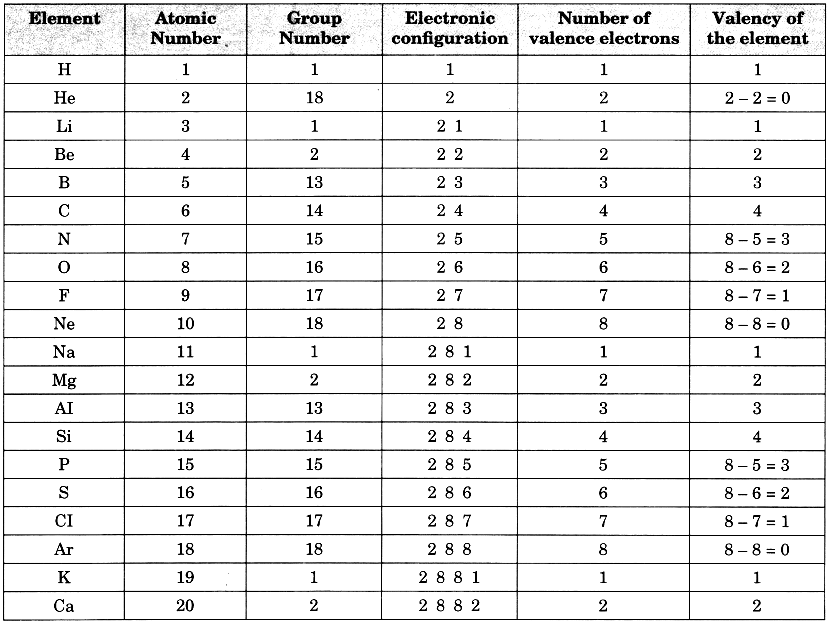• In a period, the valency first increases from 1 to 4 and then decreases from 4 to zero.
• In a group, the number of valence electrons remains the same. Therefore, all the elements in a group have the same valency. For example, the valency of all the elements of group 1 is 1, that of group 2 is 2, that of group 3 is 3 and so on.

Activity 5.7 (Textbook Page 88)

Observation:

• The decreasing order of the atomic radii of the elements of second period is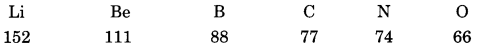• Yes, the above elements are arranged in the pattern of a period in the periodic table.
• Li has largest (152 pm) and O has the smallest (66 pm) atoms.
• The atomic radii decreases as we move from left to right in a period. This is due to increase in nuclear charge which tends to pull the electrons closer to the nucleus and hence the atomic size decreases.

Activity 5.8 (Textbook Page 89)

Observation:

• The atomic radii (in pm) of the elements of group 1 increase in the order: Li (152), Na (186), K (231), Rb (244) and Cs (262).
• Li has the smallest (152 pm) atom while Cs has the largest (262 pm) atom.
• As we go from top to bottom in a group, the atomic size increases as the number of inner filled shells increases.

Activity 5.9 (Textbook Page 89)

Observation:

• The elements of the third period from left to right are: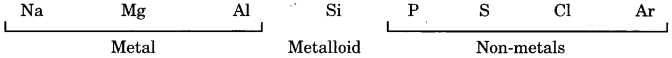• Metals lie on the left side of the Periodic Table.
• Non-metals lie on the right side of the Periodic Table.

Conclusion: Metals lie on the left side and non-metals lie on the right side of the Periodic Table.

Activity 5.10 (Textbook Page 89)

Observation:

• As we move from top to bottom in a group, the atomic size increases due to a corresponding increase in the number of inner filled shells. As a result the force of attraction of the nucleus for the electrons decreases and hence the tendency to lose electrons increases.
• As we move from left to right in a period, the nuclear charge increases regularly by one unit and hence the force of attraction of the nucleus for electrons increases. As a result the tendency to lose electrons decreases.

Conclusion: The tendency to lose electrons increases down a group but decreases across a period.

Activity 5.11 (Textbook Page 90)

Observation:

• As we go from left to right across a period, the nuclear charge increases regularly by one unit, so the force of attraction for the incoming electron increases. Consequently, the tendency to gain electrons increases across a period.
• As we go down a group, the atomic size progressively increases. As a result, the force of attraction of the nucleus for the incoming electron decreases. Consequently, the tendency to gain electrons decreases.

Conclusion: The tendency to gain electrons increases across a period and decreases down a group.